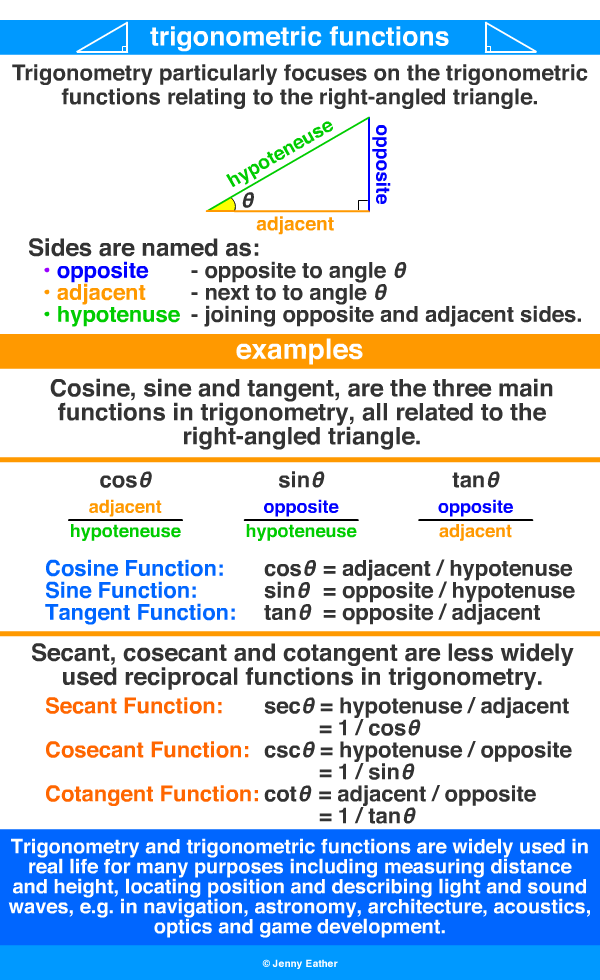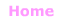Aa Bb Cc Dd Ee Ff Gg Hh Ii Jj Kk Ll Mm Nn Oo Pp Qq Rr Ss Tt Uu Vv Ww Xx Yy Zz

Tt

trigonometric functions

• trigonometric functions are defined using a right-angled triangle.
• they relate an angle of a right-angled triangle to the ratio of two side lengths.
• the most familiar trigonometric functions are cosine, sine and tangent.
• their reciprocals are respectively secant, cosecant and cotangent.

EXAMPLES:more

Introduction to Trigonometry from Math is Fun

Trigonometry functions - introduction from Math Open Reference© Jenny Eather 2014. All rights reserved.